## 2.23Summary of equations

• Force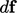at a surface: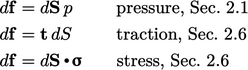• Rate of deformation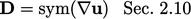### Conservation laws

• Mass, Sec. 2.4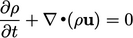• Momentum, Sec. 2.7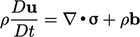• Energy, Sec. 2.15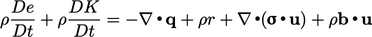### Constitutive models

• Newtonian ﬂuid, Sec. 2.12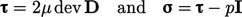• Fourier’s law, Sec. 2.16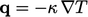• Ideal gas, Sec. 2.16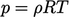• Speciﬁc heat capacity, Sec. 2.18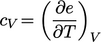• Energy-temperature relation, Sec. 2.19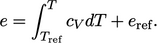### Derivatives

• Material time derivative, Sec. 2.5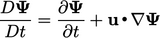• Divergence, Sec. 2.4 ,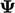is vector, tensor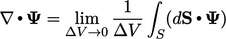• Gradient, Sec. 2.12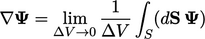• Laplacian, Sec. 2.14 ,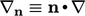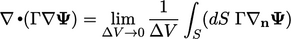Notes on CFD: General Principles - 2.23 Summary of equations